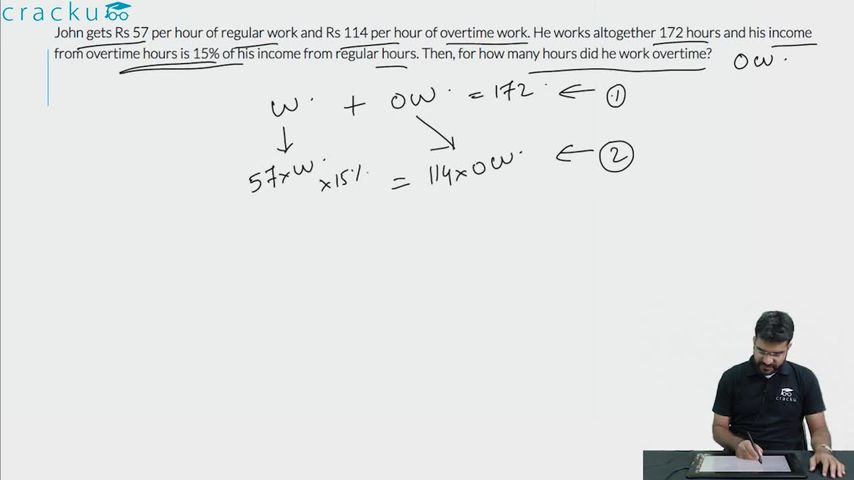Question 88

# John gets Rs 57 per hour of regular work and Rs 114 per hour of overtime work. He works altogether 172 hours and his income from overtime hours is 15% of his income from regular hours. Then, for how many hours did he work overtime?

Solution

It is given that John works altogether 172 hours i.e including regular and overtime hours.

Let a be the regular hours, 172-a will be the overtime hours

John's income from regular hours = 57*a

John's income for working overtime hours = (172-a)*144

It is given that his income from overtime hours is 15% of his income from regular hours

a*57*0.15 = (172-a)*114

a=160

The number of hours for which he worked overtime = 172-160=12 hrs

### View Video Solution